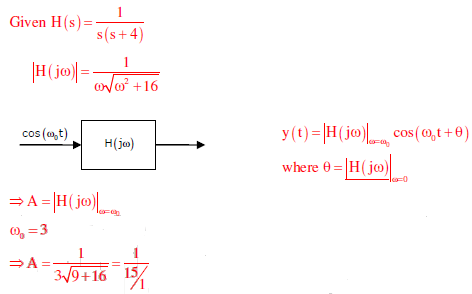# Control Systems - Online Test

Q1.
The transfer function of a compensator is given asGc(s) is a lead compensator if
Explaination / Solution: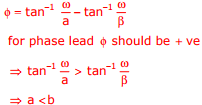both option (A) and (C) satisfier but option (C) will pot polar and zero as RHS of s-plane thus not possible option (A) is right

Q2. The transfer function of a compensator is given as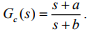The phase of the above lead compensator is maximum at
Explaination / Solution: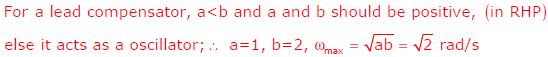Q3. The Bode plot of a transfer function G(s) is shown in the figure below.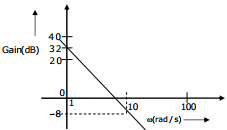The gain (20 log |G(s)|) is 32 dB and -8 dB at 1 radians/s and 10 radians/s respectively. The phase is negative for all ω.Then G(s) is
Explaination / Solution:
No Explaination.

Q4.
In the feedback network shown below, if the feedback factor k is increased, then the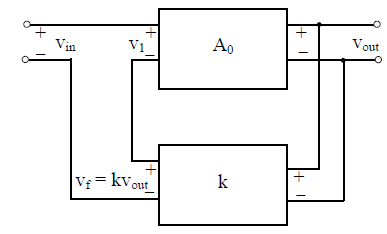Explaination / Solution:
No Explaination.

Q5. Which one of the following statements is NOT TRUE for a continuous time causal and stable LTI system?
Explaination / Solution:
No Explaination.

Q6.
Assuming zero initial condition, the response y(t) of the system given below to a unit step input u(t) is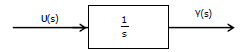Explaination / Solution:
No Explaination.

Q7. The transfer functionof the circuit shown below is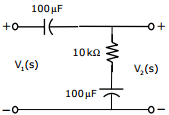Explaination / Solution:
No Explaination.

Q8. The signal flow graph for a system is given below. The Transfer function, Y(s)/U(s) for the system is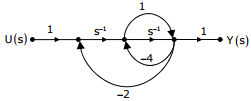Explaination / Solution:
No Explaination.

Q9. The open-loop transfer function of a dc motor is given as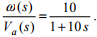When connected in feedback as shown below, the approximate value of Ka that will reduce the time constant of the closed loop system by one hundred times as compared to that of the open-loop system is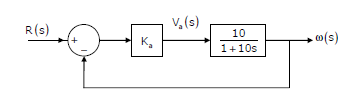Explaination / Solution:
No Explaination.

Q10. Consider an LTI system with transfer function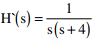If the input to the system is cos(3t) and the steady state output is Asin (3t + α), then the value of A is
Explaination / Solution: